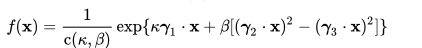# Kent Distribution: Definition

Share on

The Kent distribution, also known as the 5-parameter Fisher-Bingham distribution, is a probability distribution in ℜ3, the real three dimensional coordinate space, of a two-dimensional unit sphere.

## Kent Distribution PDF

The Kent distribution’s probability density function, f(x), is given by the equation:Here,

In the above equation, Iv(κ) represents what is called the modified Bessel function.

## References

Boomsma, W., et al. (2006) Graphical models and directional statistics capture protein structure. In S. Barber, P.D. Baxter, K.V.Mardia, & R.E. Walls (Eds.), Interdisciplinary Statistics and Bioinformatics, pp. 91–94. Leeds, Leeds University Press.
Kent, J. T. (1982) The Fisher–Bingham distribution on the sphere., J. Royal. Stat. Soc., 44:71–80.
Mardia, K. V. M., Jupp, P. E. (2000) Directional Statistics (2nd edition), John Wiley and Sons Ltd

CITE THIS AS:
Stephanie Glen. "Kent Distribution: Definition" From StatisticsHowTo.com: Elementary Statistics for the rest of us! https://www.statisticshowto.com/kent-distribution-2/
---------------------------------------------------------------------------Need help with a homework or test question? With Chegg Study, you can get step-by-step solutions to your questions from an expert in the field. Your first 30 minutes with a Chegg tutor is free!